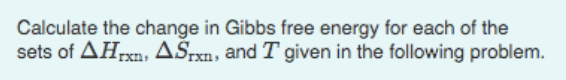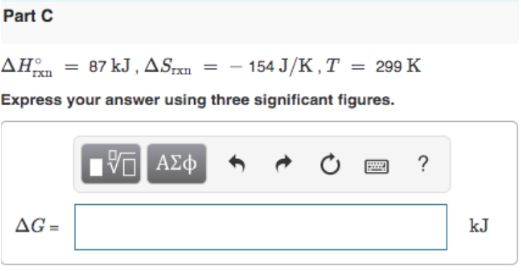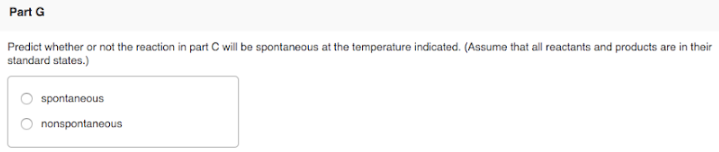# Calculate the change in Gibbs free energy for each of the sets of ΔHrxn, ΔSrxn, and T given in the following problem. ΔH°rxn = -87 kJ, ΔSrxn = -154 J/K, T = 299 K Express your answer using three significant figures. Predict whether or not the reaction in part C will be spontaneous at the temperature indicated. (Assume that all reactants and products are in their standard states.) a) spontaneous b) nonspontaneous